# How to Calculate and Solve for Acceleration | Motion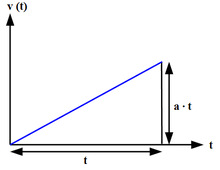The image above represents acceleration.

To compute for acceleration, three essential parameters are needed and these parameters are initial velocity (u), final velocity (v) and time (t).

The formula for calculating acceleration:

a = (v – u) / t

Where;

a = Acceleration
v = Final Velocity
u = Initial Velocity
t = Time

Let’s solve an example;
Find the acceleration when the final velocity is 21, the initial velocity is 10 and time is 8.

This implies that;

v = Final Velocity =21
u = Initial Velocity = 10
t = Time = 8

a = (v – u) / t
a = (21 – 10) / 8
a = 11 / 8
a = 1.375

Therefore, the acceleration is 1.375 m/s².

Calculating the Initial Velocity when the Acceleration, the Final Velocity and the Time is Given.

u = v – at

Where;

u = Initial Velocity
a = Acceleration
v = Final Velocity
t = Time

Let’s solve an example;
Find the initial velocity when the acceleration is 14, the final velocity is 34 and the time is 2.

This implies that;

a = Acceleration = 14
v = Final Velocity = 34
t = Time = 2

u = v – at
u = 34 – (14 x 2)
u = 34 – 28
u = 6

Therefore, the initial velocity is 6 m/s.

Calculating the Final Velocity when the Acceleration, the Initial Velocity and the Time is Given.

v = at + u

Where;

v = Final Velocity
a = Acceleration
u = Initial Velocity
t = Time

Let’s solve an example;
Find the final velocity when the acceleration is 28, the initial velocity is 12 and time is 10.

This implies that;

a = Acceleration = 28
u = Initial Velocity = 12
t = Time = 10

v = at + u
v = (28 x 10) + 12
v = 280 + 12
v = 292

Therefore, the final velocity is 292 m/s.

Calculating the Time when the Acceleration, the Initial Velocity and the Final Velocity is Given.

t = v – u / a

Where;

t = Time
a = Acceleration
v = Final Velocity
u = Initial Velocity

Let’s solve an example;
Find the time when the acceleration is 20, the initial velocity is 10 and the final velocity is 44.

This implies that;

a = Acceleration = 20
v = Final Velocity = 44
u = Initial Velocity = 10

t = v – u / a
t = 44 – 10 / 20
t = 34 / 20
t = 1.7

Therefore, the time is 1.7 s.

Nickzom Calculator – The Calculator Encyclopedia is capable of calculating the acceleration.

To get the answer and workings of the acceleration using the Nickzom Calculator – The Calculator Encyclopedia. First, you need to obtain the app.

You can get this app via any of these means:

To get access to the professional version via web, you need to register and subscribe for NGN 1,500 per annum to have utter access to all functionalities.
You can also try the demo version via https://www.nickzom.org/calculator

Apple (Paid) – https://itunes.apple.com/us/app/nickzom-calculator/id1331162702?mt=8
Once, you have obtained the calculator encyclopedia app, proceed to the Calculator Map, then click on Motion under Physics.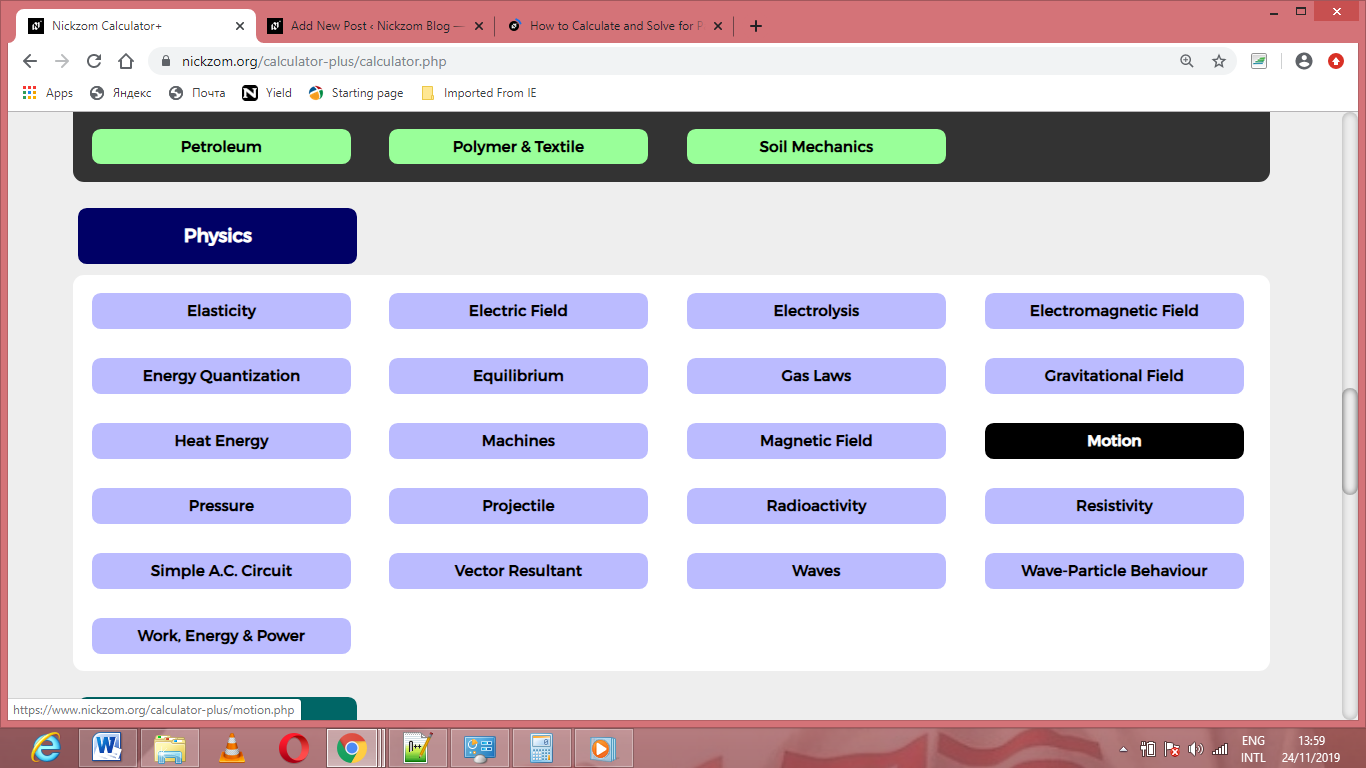Now, Click on Acceleration under Motion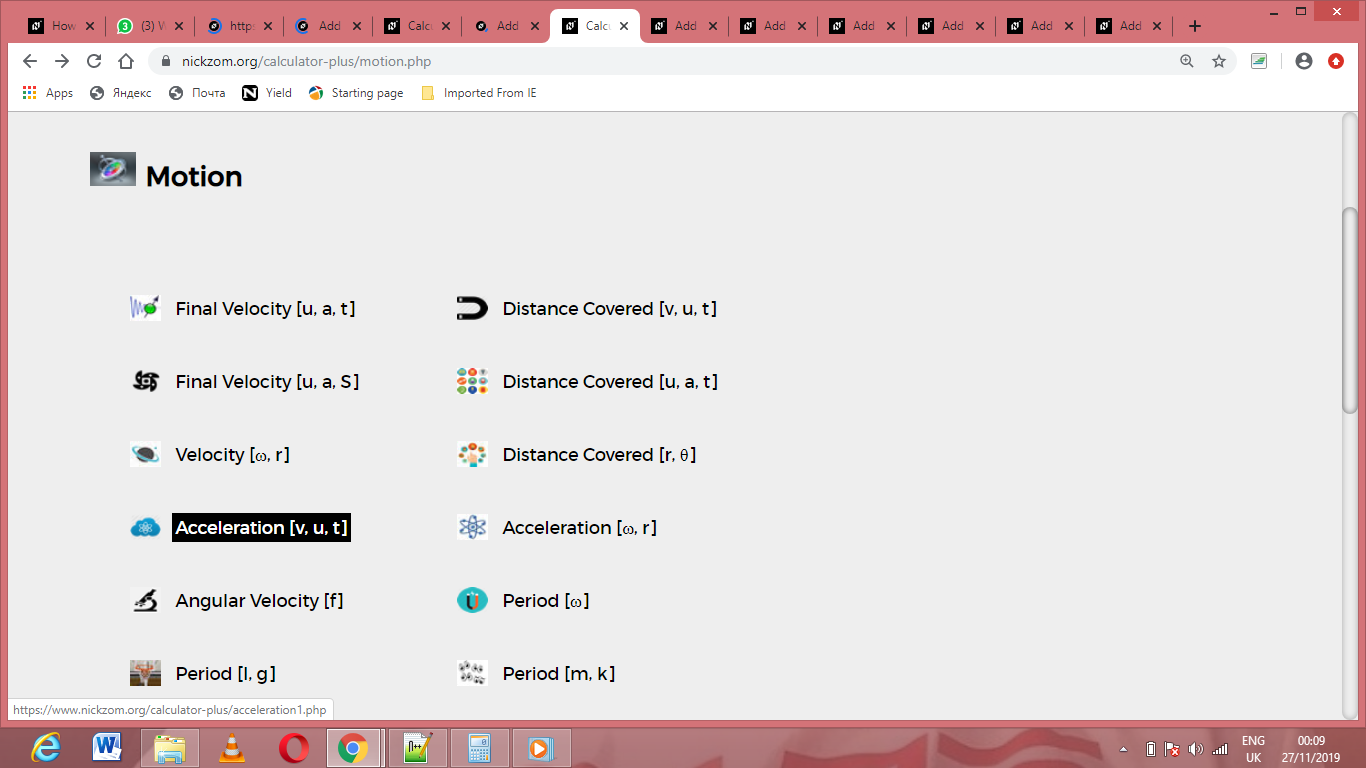The screenshot below displays the page or activity to enter your values, to get the answer for the acceleration according to the respective parameters which are the initial velocity (u), final velocity (v) and time (t).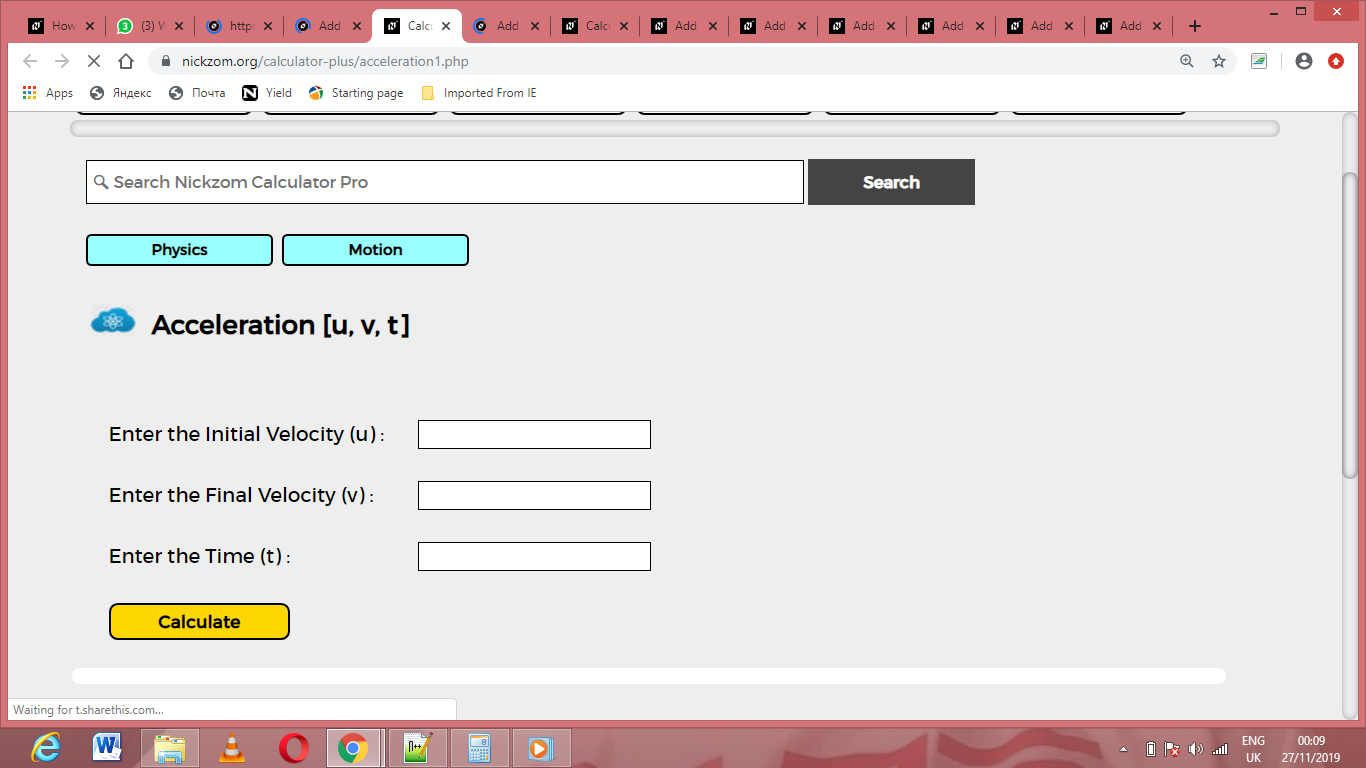Now, enter the values appropriately and accordingly for the parameters as required by the initial velocity (u) is 10, final velocity (v) is 21 and time (t) is 8.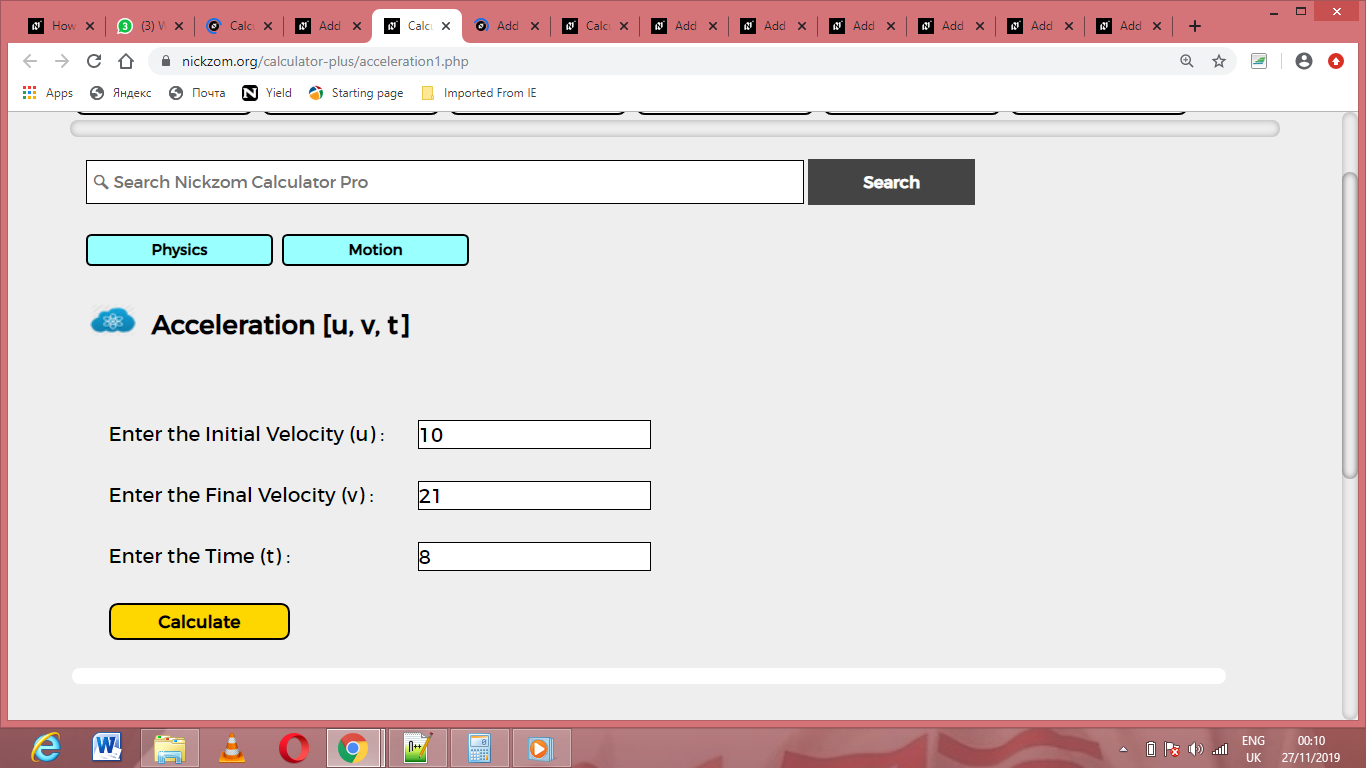Finally, Click on Calculate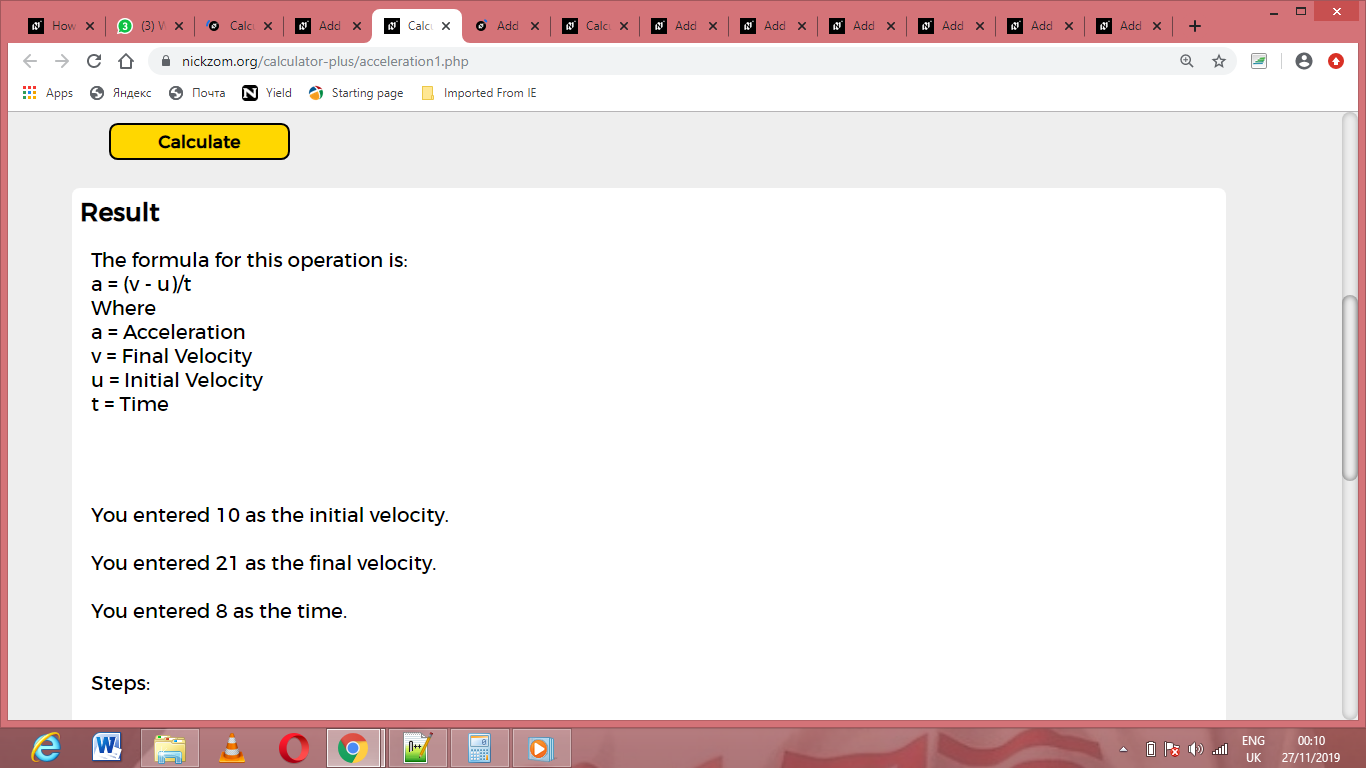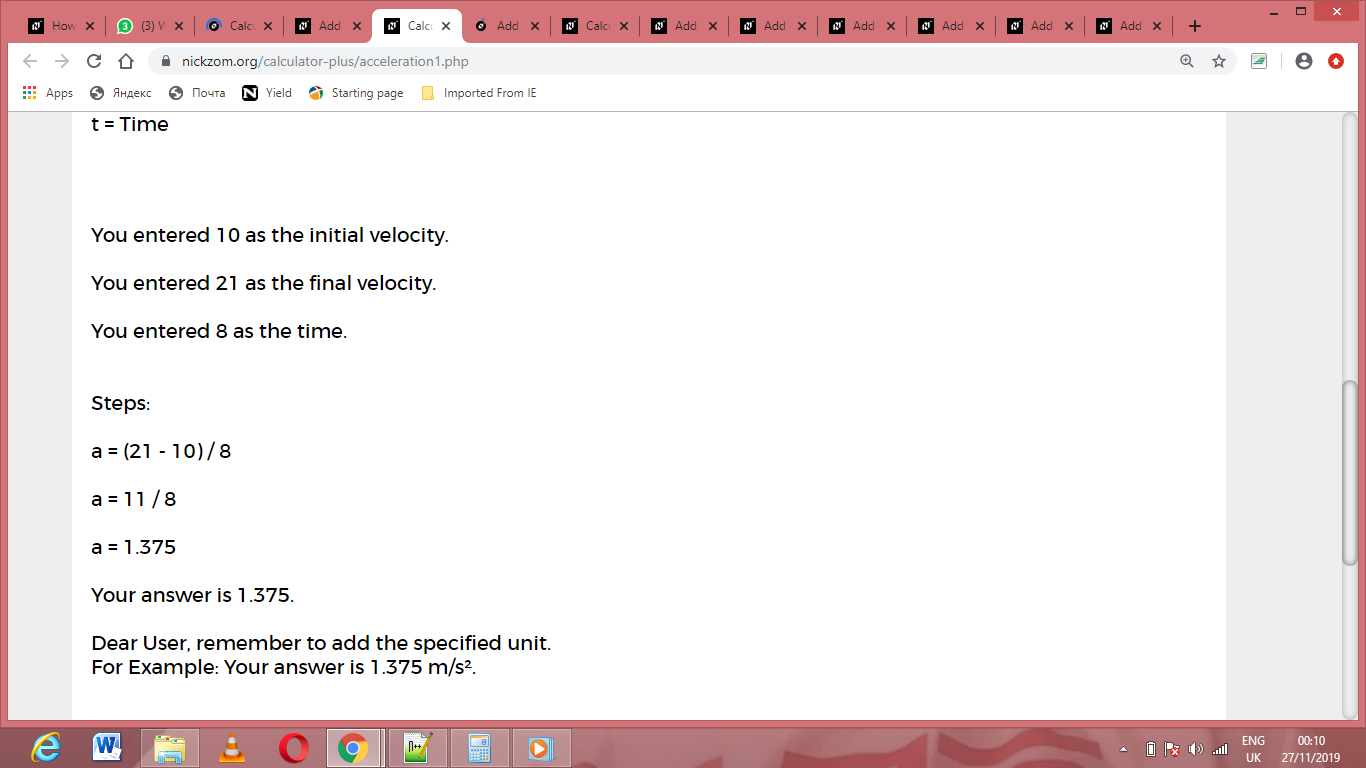As you can see from the screenshot above, Nickzom Calculator– The Calculator Encyclopedia solves for the acceleration and presents the formula, workings and steps too.